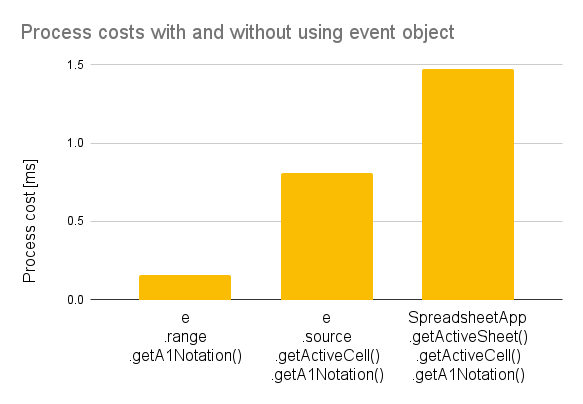Gists

# Introduction

There are event objects at Google Apps Script. Typically, users which use Spreadsheet often use onEdit(event). Here, I would like to introduce the process costs for the event objects using this onEdit(event).

When onEdit(event) is used for the spreadsheet, event of onEdit(event) has the following structure.

{
"authMode": {},
"range": {
"columnStart": 1,
"rowStart": 1,
"rowEnd": 1,
"columnEnd": 1
},
"source": {},
"oldValue": "old sample text",
"user": {
"nickname": "sampleName",
"email": "sample email"
},
"value": "sample text"
}


In this structure, for example, the range of active cell is "range": {"columnStart": 1, "rowStart": 1, "rowEnd": 1, "columnEnd": 1 }. Namely, it’s “A1”. Users can use the range of active cell using this event object. In this report, I have investigated the process cost for retrieving the range of active cell as a sample.

# Experimental procedure

The sample script is as follows.

function onEdit(e) {
var start = Date.now();
for (var i = 0; i < 5000; i++) {
var range = e.range.getA1Notation(); // A1
}
Logger.log("%s [s]", (Date.now() - start) / 1000);

var start = Date.now();
for (var i = 0; i < 5000; i++) {
var range = e.source.getActiveCell().getA1Notation(); // A1
}
Logger.log("%s [s]", (Date.now() - start) / 1000);

var start = Date.now();
for (var i = 0; i < 5000; i++) {
var range = SpreadsheetApp.getActiveSheet().getActiveCell().getA1Notation(); // A1
}
Logger.log("%s [s]", (Date.now() - start) / 1000);
}


In this sample script, the range of active cell is retrieved for 5000 times, and the time is measured. In this experiment, the average values of 10 times were used as the result.

## Resultsmethod Time in 5000 times [s] Time in 1 times [ms]
e.range.getA1Notation() 0.784 0.157
e.source.getActiveCell().getA1Notation() 4.048 0.810
From above table, it was found that the process cost of e.range.getA1Notation() was 20 % and 10 % for those of e.source.getActiveCell().getA1Notation() and SpreadsheetApp.getActiveSheet().getActiveCell().getA1Notation(), respectively. It is considered that these process costs depend on the number of use of APIs. As the result, it was found that the event objects can decrease the process cost in the scripts. Although this is the general result, also I think that it is important to quantitatively know this.